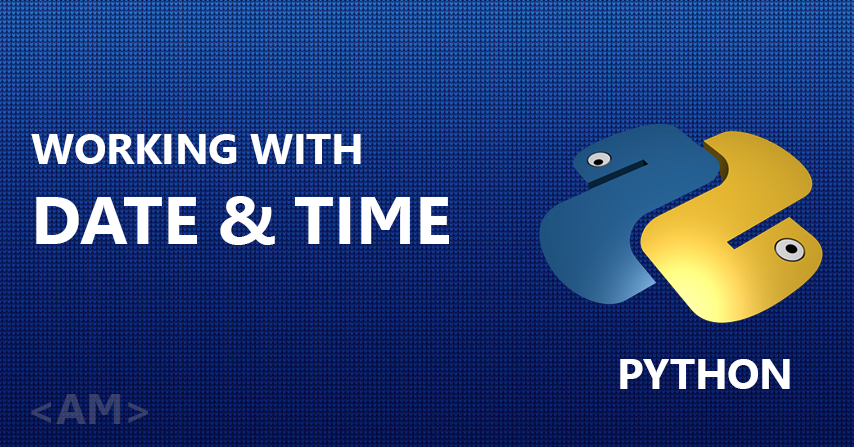February 12, 2019

# Working with date and time in PythonLike every programming language, python has got some predefined libraries to import functions from and use them in your code. The most generic of which is date.

We will start with importing the predefined library for using dates and related functions in our program. Today’s date is 12th of february 2019

```import datetime
```

This will import the library. Now using this library we can use the below functions

`currentDate = datetime.date.today()`

Storing the current date inside the CurrentDate variable.

`print(currentDate)`

Output : 2019-02-12

`print(currentDate.year) #year is propety of currentDate object`

Output : 2019

`print(currentDate.month) #month is propety of currentDate object`

Output : 2

`print(currentDate.day) #day is propety of currentDate object`

Output : 12

## Date Format

We can change the way the date is being displayed.

the default format is YYYY-MM-DD

For printing something like 12 Feb, 2019, we use

`print(currentDate.strftime('%d %b,%Y '))`

function strftime() – converts time to string format.
Where,

%d = Date ,
%b = First 3 characters of the month,
%Y = the year.

Wish to know all formats ? have a look at string from time reference.

So here is a small program to calculate the month of your birthday from a given input.

```birthday = input("What is your birthday?")
birthday = datetime.datetime.strptime(birthday,"%d/%m/%Y").date() #**

We ask the user to input his birthday | input : 04/01/1993
Output: Your birthday is in January

**Why we used datetime 2 times ?

Just because we need to call the function strptime which is in the datetime module.

Calculating Days between today and your next birthday.

```import datetime
```import datetime